# Calculus symbols

## Calculus & analysis math symbols table

Symbol Symbol Name Meaning / definition Example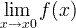limit limit value of a function
ε epsilon represents a very small number, near zero ε 0
e e constant / Euler's number e = 2.718281828... e = lim (1+1/x)x , x→∞
y ' derivative derivative - Lagrange's notation (3x3)' = 9x2
y '' second derivative derivative of derivative (3x3)'' = 18x
y(n) nth derivative n times derivation (3x3)(3) = 18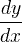derivative derivative - Leibniz's notation d(3x3)/dx = 9x2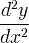second derivative derivative of derivative d2(3x3)/dx2 = 18x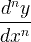nth derivative n times derivation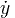time derivative derivative by time - Newton's notation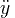time second derivative derivative of derivative
Dx y derivative derivative - Euler's notation
Dx2y second derivative derivative of derivative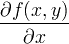partial derivative   ∂(x2+y2)/∂x = 2x
integral opposite to derivation
double integral integration of function of 2 variables
triple integral integration of function of 3 variables
closed contour / line integral
closed surface integral
closed volume integral
[a,b] closed interval [a,b] = {x | a x b}
(a,b) open interval (a,b) = {x | a < x < b}
i imaginary unit i ≡ √-1 z = 3 + 2i
z* complex conjugate z = a+biz*=a-bi z* = 3 + 2i
z complex conjugate z = a+biz = a-bi z = 3 + 2i
Re(z) real part of a complex number z = a+bi → Re(z)=a Re(3 - 2i) = 3
Im(z) imaginary part of a complex number z = a+bi → Im(z)=b Im(3 - 2i) = -2
| z | absolute value/magnitude of a complex number |z| = |a+bi| = √(a2+b2) |3 - 2i| = √13
arg(z) argument of a complex number The angle of the radius in the complex plane arg(3 + 2i) = 33.7°
nabla / del gradient / divergence operator f (x,y,z)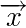vector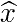unit vector
x * y convolution y(t) = x(t) * h(t)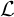Laplace transform F(s) ={f (t)}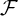Fourier transform X(ω) ={f (t)}
δ delta function
lemniscate infinity symbol

Currently, we have around 1976 calculators, conversion tables and usefull online tools and software features for students, teaching and teachers, designers and simply for everyone.